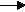gcsescience.com                                       7                                       gcsescience.com

Oxidation and Reduction.

What is Oxidation and What is Reduction?

Oxidation means losing electrons
and reduction means gaining electrons.

In the reaction between iron and copper(II) sulfate

Fe(s)  +  Cu2+(aq)Fe2+(aq)  +  Cu(s)

Iron metal (Fe(s)) loses 2 electrons to form iron ions (Fe2+(aq))
Fe(s)  -  2e-(aq)Fe2+(aq)
This is called oxidation. Iron is said to be oxidised.

Copper ions (Cu2+(aq)) gain 2 electrons to form copper metal (Cu(s))
Cu2+(aq)  +  2e-(aq)Cu(s)
This is called reduction. Copper is said to be reduced.

and reduction means gaining electrons,
remember  O I L R I G
(Oxidation Is Loss, Reduction Is Gain - of electrons).

Oxidation and reduction always occur together.
They are called redox reactions
(pronounced "reed - ox" for reduction / oxidation).

Oxidation may also be defined as gaining oxygen
and reduction defined as losing oxygen.
For example, when copper(II) oxide is heated with carbon

copper(II) oxide + carboncopper + carbon dioxide.
2CuO(s)      +     C(s)2Cu(s)     +    CO2(g)

Carbon is oxidised by gaining oxygen to form carbon dioxide.
Copper (in CuO) is reduced by losing oxygen to form
copper metal.
Note that copper has changed from Cu2+ (in CuO) to Cu(s)
and so has gained electrons.
Cu2+(aq)  +  2e-(aq)Cu(s)

gcsescience.com    The Periodic Table    Index    Reactivity Series Quiz    gcsescience.com